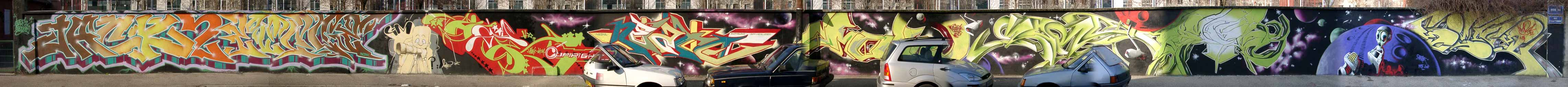J A C K   T W O   C I A   /   E V A D   P V   /   I M   C R E W   /   N E S T A   B O T H S I D E   H O T   /   M O S H   B O T H S I D E   /   K O R E M   B O T H S I D E   /   B R U S K   K M F  G R E N O B L E   2 0 0 1